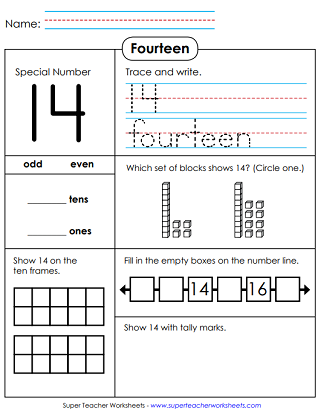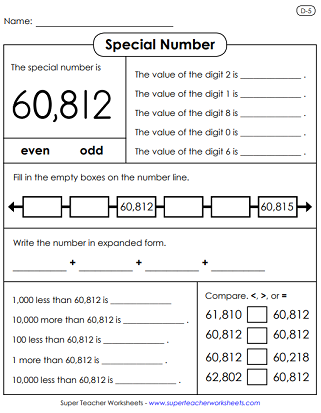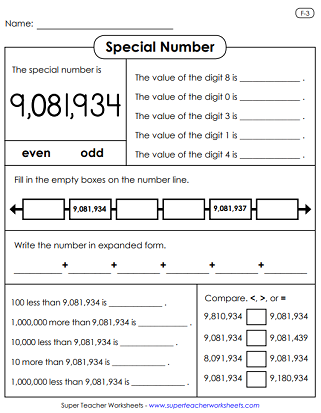# Special Number Worksheets

This page has special number worksheets for 1, 2, 3, 4, and 5-digit numbers. For each number, students determine whether the special number is odd or even, state the values of each digit, and write the number in expanded form.## Single-Digit Numbers

Color one lion. Show 1 on the ten frame. Circle the number ones. Trace the letters in the word one.
Fill in the 10-frame to show 2. Circle the 2s. Color two delicious apples in the array.
Trace and print the word three. Use crayons to color three spiral seashells in the illustration. Write the numbers that come before and after 3.
Count the whales and shade four of them. Write the number that comes between 2 and 3. Write the number that comes between 3 and 4. Circle the digit 4 in the illustration.
Do you see 5 baseballs in the picture? Show the number 5 on a ten frame. Circle the 5s but do not circle the letter S.
Ribbit. Ribbit. Use a green crayon to color six frogs. Then trace the letters s-i-x. Then write the number that comes between 4 and 6.
Lucky seven! Can your kids count seven cars? Then circle all of the sevens in the letter-number box.
Color eight pens. Show 8 with tallies. Circle the 8s in the illustration.
Shade in 9 squares on the array. Count nine wooden pencils. Trace the letters n-i-n-e and the digit 9.

## 2-Digit Numbers

Show 10 with tally marks. Circle the tens in the box. Trace and write the number ten.
Trace the digits in the number eleven. Trace the letters to make the word eleven. Make 11 dots on the ten frames. Choose the P.V. blocks for 11.
Tell whether 12 is even or odd. Circle 12 place value blocks. Make 12 on the 10-frame grid and show 12 with tallies.
Unlucky 13! Choose the P.V. blocks that represent 13. Tell if it is odd or even. Mark 13 squares on the array.
For this one, students trace the letters f-o-u-r-t-e-e-n. Count and mark 14 squares in the array. Show the special number with tallies.
Learn all about the very special number fifteen! Kids can answer the place value questions related to this number.
The S.N. worksheet for sixteen is much like the worksheets for the other numbers. PlaceVal cubes, number line, tallies.
Seventeen is a very special number, wouldn't you agree? On this worksheet, kids have to look closely at P.V. cubes to figure out which one is 17. They also have to trace the word seventeen. Complete the number line and draw 17 tally marks.
Use the 10-frame array to make the number 18. Trace and write the number 18, and the word eighteen. Then finish the number line.
If 19 is your number of the day, print this place value worksheet and use it with your students.
The number twenty if the focus of this worksheet. How many tens are in the number twenty? How many ones? Can you complete the number line and show what comes before and after this number?

## 3-Digit Numbers

Show the set of place value blocks represents 349. Write 349 in expanded form. State how many 100s, 10s, and 1s are in the special number.
Write 506 in expanded form. Then tell the value of each digit. Complete the number line to tell what comes before and after 506.
Use "greater than" and "less than" symbols to compare 123 to other numbers. Complete the number line. Tell how many hundreds, tens, and ones.
Determine whether the number 780 is odd or even. Choose the set of blocks that represent 780. How much is 10 more and 10 less than 780?
Then tell what 100 more and 100 less than 467 equals. Tell how many 100s, 10s, and 1s are in 467. Complete the number line to show numbers that come after and before 467.
Write the values of the digits in 201. Write the special number in expanded form. Compare using the symbols <, >, and =.
On this worksheet, students will answer a set of questions pertaining to the number six hundred thirty-four. The will tell whether this number is even or odd. Add and subtract using this number. Find the number using place value blocks.
Write 810 in expanded form. Then complete the number line. Tell which P.V. blocks show 810. Determine if 810 is even or odd.
Which block set makes 952? Is 952 odd or even? Complete the number line. Tell how many 100s, 10s, and 1s.
This special number is unique because it has 0 tens and 0 ones.

## 4-Digit Numbers

For this special number, students must give the value of the digits 5, 7, and 1. Then circle the set of PlaceVal cubes that show 1,507. After that, they add 100 and 1,000 to this number.
Write this number in expanded form, like this: 2,000+300+50+4. Then calculate 10 less than 2,354. And find 1,000 more than 2,354.
Compare 4,092 with other numbers using the symbols <, >, and =. Tell whether or not the P.V. cubes show 4,092. Find a hundred more and a hundred less than 4,092.
The special number on this worksheet is six thousand, eight hundred twenty. Students answer a set of place value questions.
Students will answer p.v. questions to go along with three thousand, one hundred twenty-six. Includes value of the digits, even/odd, number line, and exp. form.
This one might be a little trickier for students because it's a four-digit number with only two non-zero digits.
This special number worksheet is much like the others. It'll ask kids to determine the value of each digit, complete the number line, add, subtract, and write the number as an expanded addition problem.
The s.n. for this worksheet is two thousand, three. Complete each box by filling in information about PlaceVal blocks, expanded notation, and the values of the digits.
Draw a ring around the place value blocks that show three thousand, forty-nine. Finish the incomplete number line. Write the value of the digits.

## 5-Digit Numbers

State the value of each digit, write the number in expanded form, and compare numbers. Also, write the number that is 10,000 less.
On this worksheet, students will answer a series of problems and questions about the place values in the number thirty thousand, eight hundred forty.
Find the values of the digits 1, 9, and 5. Compare using symbols for greater than, less than, and equal to. Write the number in expanded form. Tell if the number is even or if it is odd.
Answer the place-val questions relating to the number forty-three thousands and seven.
Kids must tell whether the number is odd or even. Complete the number line by telling which numbers come before and after this number. Subtract 10,000 and add 1,000.
The questions on this S.N. activity sheet center around the number seventy-six thousand, one hundred thirty-four.
Answer place value questions pertaining to the number ninety-eight thousand, seven hundred sixty-five.
Complete the number line, compare, and write the values of the digits. Also, perform basic operations by adding and subtracting ten, one hundred, and one thousand.
The special number is 59,703. Students will tell whether the number is even or odd. Write the number in expanded form. Subtract from and add to this number. And compare using <, >, and =.

## 6-Digit Numbers

Answer several place value questions for the number 304,962. Give the values of the digits. Write the number in expanded form. Add and subtract with this number.
Subtract from the special number. Add to the special number. Compare with other numbers. Complete the number line and write in expanded form.
This worksheet has many questions relating to the number nine hundred eighty-seven thousand, sixty five.
Here's another special number worksheet. This time, students will be working with the number five hundred sixty-four thousand, three hundred.
State the values of the digits in this number. Determine whether it is even or odd. Add 100 and 10,000 to this number. Compare this number to other numbers.
The S.N. on this worksheet is six hundred thirty-two thousand, fifteen.
This one is similar to the others. There's a number line, an expanded form question, comparing, adding, subtracting, and digit values.
Once again, student find digit values, compare the special number to other numbers, complete the number line, and write in expanded form.
This activity page has P.V. questions related to the number seven hundred forty-five thousand, six hundred two.

## 7-Digit Numbers

On this worksheet, students will write the values of each digit in the special number. Then, tell if it's odd or even. Then, use the symbols <, >, and = to compare to other numbers. And they'll write it in expanded form.
This activity sheet focuses on the number one million, three hundred ninety-two thousand, eight hundred seventy.
This is the largest special number so far! Nine million, eighty-one thousand, nine hundred thirty-four. Answer many place value concept questions.
Complete the number line, add and subtract, compare, and list the digit values.
On this page, students will answer questions for the number: eight million, three hundred fifty-one thousand, two hundred seven.
Place Value Worksheets

Navigate to resources for teaching place value of 2, 3, 4, 5, 6, and 7-digit numbers. Includes classroom games, fun activities, and worksheets.

Numbers and Counting (Up to 30)

Trace and print numbers, and basic counting up to 10, 20, and 30.

## Sample Worksheet ImagesMy Account
Site Information